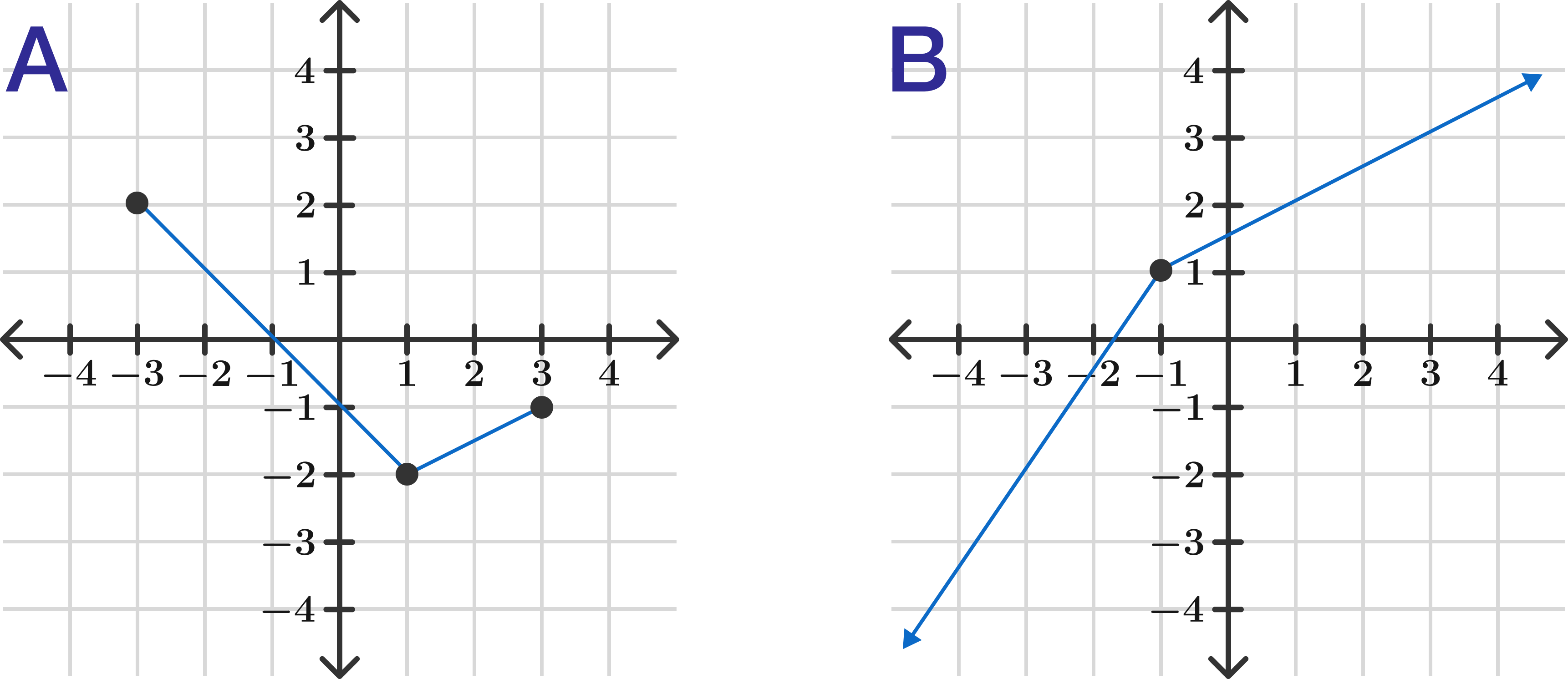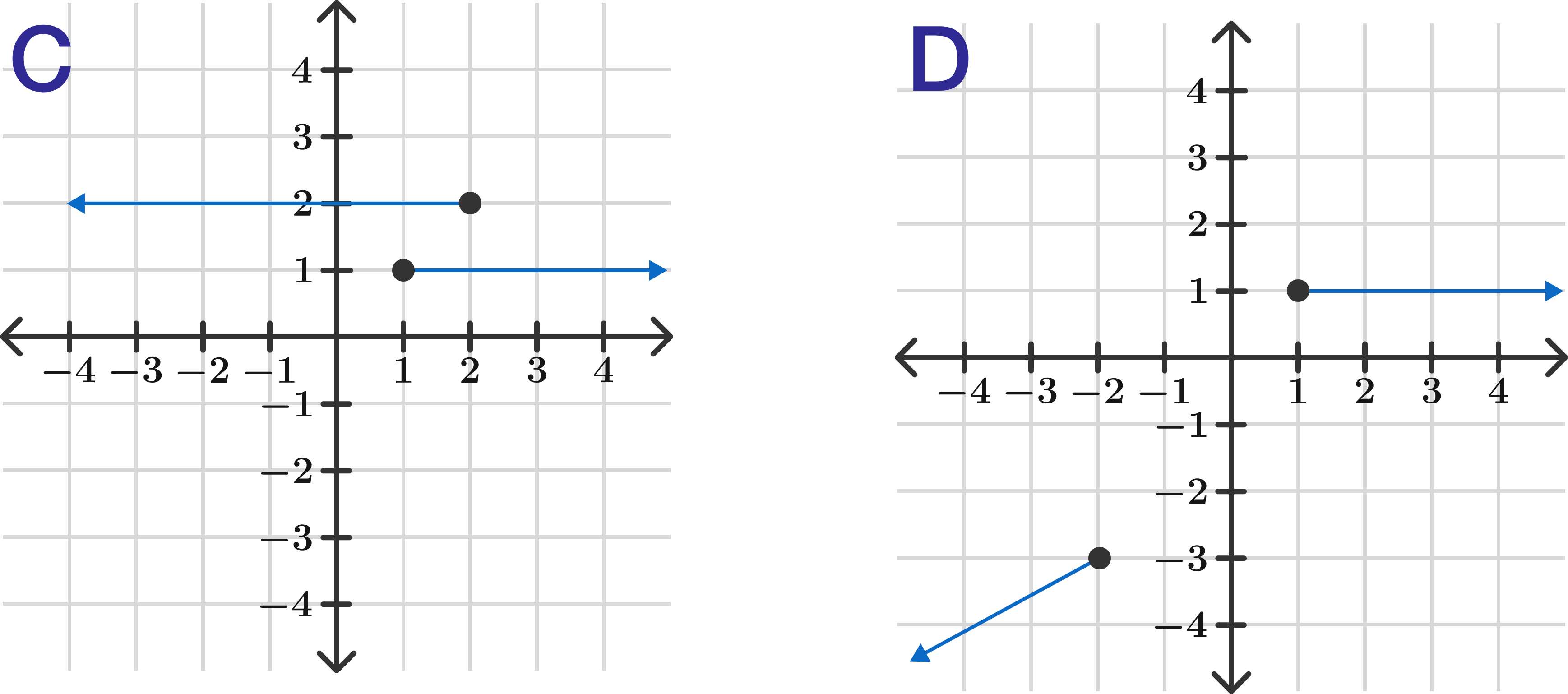Algebra II

Welcome to Modeling and Functions! This course is at the level after Algebra 1 and starts where the Algebra Fundamentals course left off.

If you see a graph with sliders underneath, that means the sliders can be moved around to help solve the problem. Try moving the sliders below this graph!

Modeling and Functions

The graph below shows world record times at running a mile from 1900 to 1960, as tracked by the International Association of Athletics Federations. The $x$-axis shows years where $0$ is 1900; the $y$-axis shows times in seconds. Based on the data shown in the graph, and assuming the model is linear, approximately what should the world record at the mile have been in 2000?

Modeling and Functions

The graph below shows three snapshots of a model rocket (rocket not to scale) being launched at an angle. The $x$-axis shows horizontal distance in meters and the $y$-axis shows vertical distance in meters. Approximately how far away would you expect the rocket to land from its starting point?

Assume the rocket will travel in a parabolic path (without wind or other considerations). Both the exact end and tip of each rocket must be part of the final curve

Modeling and Functions

The previous problem depicted a function, because it related two sets of numbers (distance and height) such that if you checked any valid distance, the rocket would be at one (and only one) specific height. The distance in this case is the input of the function and the height is the output.

Formally, we can say the height is a function of the distance.

Is the reverse true, that the distance is a function of the height?

Modeling and Functions

Functions are processes that take some input and produce some output, where every valid input produces one specific output.

If inputs are on the horizontal $x$-axis and outputs are on the vertical $y$-axis on the graphs below, how many of them are functions?Modeling and Functions

The domain of a function is the set of all valid inputs.

The below graph is of $f(x) = \sqrt{x^2 - a} .$ For which value of $a$ is the domain of $f(x)$ in the regions $x \leq -4$ and $x \geq 4 ?$

(Assume we are using real numbers only.)

Modeling and Functions

Functions are quite powerful in that they let us prove general things that apply to all graphs, even if we are uncertain what those graphs are.

In the graph below, you can change a base function $f(x)$ with a value of $Z.$ Which function corresponds with the situation?

×